# sklearn.linear_model.RidgeCV¶

class sklearn.linear_model.RidgeCV(alphas=(0.1, 1.0, 10.0), *, fit_intercept=True, scoring=None, cv=None, gcv_mode=None, store_cv_values=False, alpha_per_target=False)[source]

Ridge regression with built-in cross-validation.

See glossary entry for cross-validation estimator.

By default, it performs efficient Leave-One-Out Cross-Validation.

Read more in the User Guide.

Parameters:
alphasarray-like of shape (n_alphas,), default=(0.1, 1.0, 10.0)

Array of alpha values to try. Regularization strength; must be a positive float. Regularization improves the conditioning of the problem and reduces the variance of the estimates. Larger values specify stronger regularization. Alpha corresponds to 1 / (2C) in other linear models such as LogisticRegression or LinearSVC. If using Leave-One-Out cross-validation, alphas must be positive.

fit_interceptbool, default=True

Whether to calculate the intercept for this model. If set to false, no intercept will be used in calculations (i.e. data is expected to be centered).

scoringstr, callable, default=None

A string (see model evaluation documentation) or a scorer callable object / function with signature scorer(estimator, X, y). If None, the negative mean squared error if cv is ‘auto’ or None (i.e. when using leave-one-out cross-validation), and r2 score otherwise.

cvint, cross-validation generator or an iterable, default=None

Determines the cross-validation splitting strategy. Possible inputs for cv are:

• None, to use the efficient Leave-One-Out cross-validation

• integer, to specify the number of folds.

• An iterable yielding (train, test) splits as arrays of indices.

For integer/None inputs, if y is binary or multiclass, StratifiedKFold is used, else, KFold is used.

Refer User Guide for the various cross-validation strategies that can be used here.

gcv_mode{‘auto’, ‘svd’, ‘eigen’}, default=’auto’

Flag indicating which strategy to use when performing Leave-One-Out Cross-Validation. Options are:

'auto' : use 'svd' if n_samples > n_features, otherwise use 'eigen'
'svd' : force use of singular value decomposition of X when X is
dense, eigenvalue decomposition of X^T.X when X is sparse.
'eigen' : force computation via eigendecomposition of X.X^T


The ‘auto’ mode is the default and is intended to pick the cheaper option of the two depending on the shape of the training data.

store_cv_valuesbool, default=False

Flag indicating if the cross-validation values corresponding to each alpha should be stored in the cv_values_ attribute (see below). This flag is only compatible with cv=None (i.e. using Leave-One-Out Cross-Validation).

alpha_per_targetbool, default=False

Flag indicating whether to optimize the alpha value (picked from the alphas parameter list) for each target separately (for multi-output settings: multiple prediction targets). When set to True, after fitting, the alpha_ attribute will contain a value for each target. When set to False, a single alpha is used for all targets.

New in version 0.24.

Attributes:
cv_values_ndarray of shape (n_samples, n_alphas) or shape (n_samples, n_targets, n_alphas), optional

Cross-validation values for each alpha (only available if store_cv_values=True and cv=None). After fit() has been called, this attribute will contain the mean squared errors if scoring is None otherwise it will contain standardized per point prediction values.

coef_ndarray of shape (n_features) or (n_targets, n_features)

Weight vector(s).

intercept_float or ndarray of shape (n_targets,)

Independent term in decision function. Set to 0.0 if fit_intercept = False.

alpha_float or ndarray of shape (n_targets,)

Estimated regularization parameter, or, if alpha_per_target=True, the estimated regularization parameter for each target.

best_score_float or ndarray of shape (n_targets,)

Score of base estimator with best alpha, or, if alpha_per_target=True, a score for each target.

New in version 0.23.

n_features_in_int

Number of features seen during fit.

New in version 0.24.

feature_names_in_ndarray of shape (n_features_in_,)

Names of features seen during fit. Defined only when X has feature names that are all strings.

New in version 1.0.

Ridge

Ridge regression.

RidgeClassifier

Classifier based on ridge regression on {-1, 1} labels.

RidgeClassifierCV

Ridge classifier with built-in cross validation.

Examples

>>> from sklearn.datasets import load_diabetes
>>> from sklearn.linear_model import RidgeCV
>>> clf = RidgeCV(alphas=[1e-3, 1e-2, 1e-1, 1]).fit(X, y)
>>> clf.score(X, y)
0.5166...


Methods

 fit(X, y[, sample_weight]) Fit Ridge regression model with cv. Get metadata routing of this object. get_params([deep]) Get parameters for this estimator. Predict using the linear model. score(X, y[, sample_weight]) Return the coefficient of determination of the prediction. set_fit_request(*[, sample_weight]) Request metadata passed to the fit method. set_params(**params) Set the parameters of this estimator. set_score_request(*[, sample_weight]) Request metadata passed to the score method.
fit(X, y, sample_weight=None)[source]

Fit Ridge regression model with cv.

Parameters:
Xndarray of shape (n_samples, n_features)

Training data. If using GCV, will be cast to float64 if necessary.

yndarray of shape (n_samples,) or (n_samples, n_targets)

Target values. Will be cast to X’s dtype if necessary.

sample_weightfloat or ndarray of shape (n_samples,), default=None

Individual weights for each sample. If given a float, every sample will have the same weight.

Returns:
selfobject

Fitted estimator.

Notes

When sample_weight is provided, the selected hyperparameter may depend on whether we use leave-one-out cross-validation (cv=None or cv=’auto’) or another form of cross-validation, because only leave-one-out cross-validation takes the sample weights into account when computing the validation score.

Get metadata routing of this object.

Please check User Guide on how the routing mechanism works.

Returns:

A MetadataRequest encapsulating routing information.

get_params(deep=True)[source]

Get parameters for this estimator.

Parameters:
deepbool, default=True

If True, will return the parameters for this estimator and contained subobjects that are estimators.

Returns:
paramsdict

Parameter names mapped to their values.

predict(X)[source]

Predict using the linear model.

Parameters:
Xarray-like or sparse matrix, shape (n_samples, n_features)

Samples.

Returns:
Carray, shape (n_samples,)

Returns predicted values.

score(X, y, sample_weight=None)[source]

Return the coefficient of determination of the prediction.

The coefficient of determination $$R^2$$ is defined as $$(1 - \frac{u}{v})$$, where $$u$$ is the residual sum of squares ((y_true - y_pred)** 2).sum() and $$v$$ is the total sum of squares ((y_true - y_true.mean()) ** 2).sum(). The best possible score is 1.0 and it can be negative (because the model can be arbitrarily worse). A constant model that always predicts the expected value of y, disregarding the input features, would get a $$R^2$$ score of 0.0.

Parameters:
Xarray-like of shape (n_samples, n_features)

Test samples. For some estimators this may be a precomputed kernel matrix or a list of generic objects instead with shape (n_samples, n_samples_fitted), where n_samples_fitted is the number of samples used in the fitting for the estimator.

yarray-like of shape (n_samples,) or (n_samples, n_outputs)

True values for X.

sample_weightarray-like of shape (n_samples,), default=None

Sample weights.

Returns:
scorefloat

$$R^2$$ of self.predict(X) w.r.t. y.

Notes

The $$R^2$$ score used when calling score on a regressor uses multioutput='uniform_average' from version 0.23 to keep consistent with default value of r2_score. This influences the score method of all the multioutput regressors (except for MultiOutputRegressor).

set_fit_request(*, sample_weight: Union[bool, None, str] = '$UNCHANGED$') [source]

Request metadata passed to the fit method.

Note that this method is only relevant if enable_metadata_routing=True (see sklearn.set_config). Please see User Guide on how the routing mechanism works.

The options for each parameter are:

• True: metadata is requested, and passed to fit if provided. The request is ignored if metadata is not provided.

• False: metadata is not requested and the meta-estimator will not pass it to fit.

• None: metadata is not requested, and the meta-estimator will raise an error if the user provides it.

• str: metadata should be passed to the meta-estimator with this given alias instead of the original name.

The default (sklearn.utils.metadata_routing.UNCHANGED) retains the existing request. This allows you to change the request for some parameters and not others.

New in version 1.3.

Note

This method is only relevant if this estimator is used as a sub-estimator of a meta-estimator, e.g. used inside a Pipeline. Otherwise it has no effect.

Parameters:
sample_weightstr, True, False, or None, default=sklearn.utils.metadata_routing.UNCHANGED

Metadata routing for sample_weight parameter in fit.

Returns:
selfobject

The updated object.

set_params(**params)[source]

Set the parameters of this estimator.

The method works on simple estimators as well as on nested objects (such as Pipeline). The latter have parameters of the form <component>__<parameter> so that it’s possible to update each component of a nested object.

Parameters:
**paramsdict

Estimator parameters.

Returns:
selfestimator instance

Estimator instance.

set_score_request(*, sample_weight: Union[bool, None, str] = '$UNCHANGED$') [source]

Request metadata passed to the score method.

Note that this method is only relevant if enable_metadata_routing=True (see sklearn.set_config). Please see User Guide on how the routing mechanism works.

The options for each parameter are:

• True: metadata is requested, and passed to score if provided. The request is ignored if metadata is not provided.

• False: metadata is not requested and the meta-estimator will not pass it to score.

• None: metadata is not requested, and the meta-estimator will raise an error if the user provides it.

• str: metadata should be passed to the meta-estimator with this given alias instead of the original name.

The default (sklearn.utils.metadata_routing.UNCHANGED) retains the existing request. This allows you to change the request for some parameters and not others.

New in version 1.3.

Note

This method is only relevant if this estimator is used as a sub-estimator of a meta-estimator, e.g. used inside a Pipeline. Otherwise it has no effect.

Parameters:
sample_weightstr, True, False, or None, default=sklearn.utils.metadata_routing.UNCHANGED

Metadata routing for sample_weight parameter in score.

Returns:
selfobject

The updated object.

## Examples using sklearn.linear_model.RidgeCV¶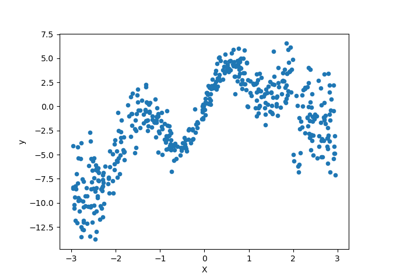Combine predictors using stacking

Combine predictors using stacking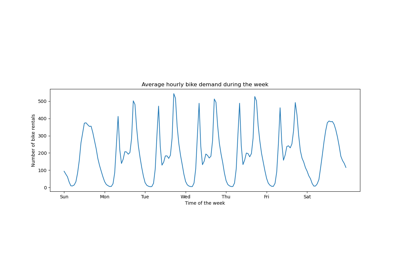Time-related feature engineering

Time-related feature engineering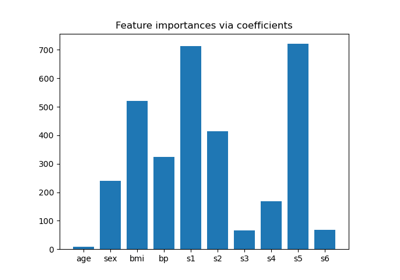Model-based and sequential feature selection

Model-based and sequential feature selection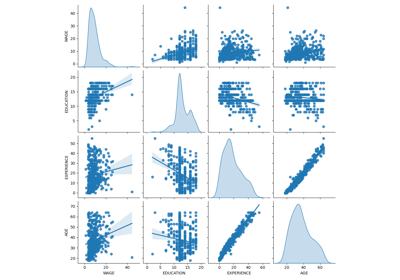Common pitfalls in the interpretation of coefficients of linear models

Common pitfalls in the interpretation of coefficients of linear models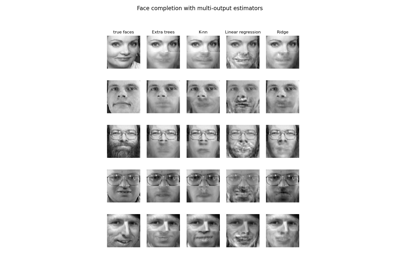Face completion with a multi-output estimators

Face completion with a multi-output estimators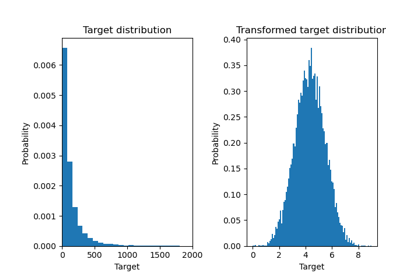Effect of transforming the targets in regression model

Effect of transforming the targets in regression model